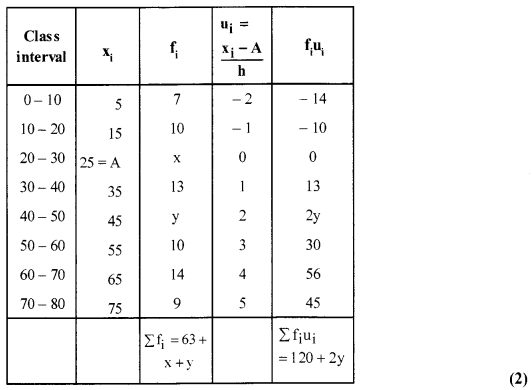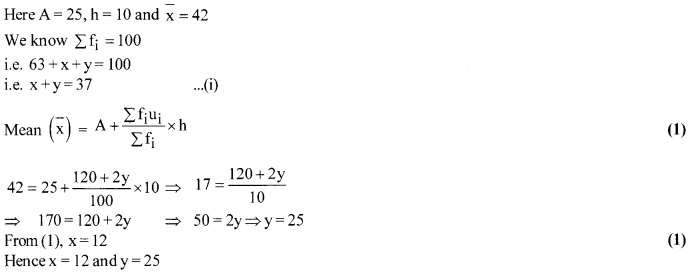# CBSE Sample Paper for Class 10th Mathematics Paper 06 | CBSE Exam | CBSE Notes

Here we are giving a sample paper to all of you CBSE board students, through which all of you can score very well in your exams. In this post, we are giving you a sample paper of class 10 maths. hope you benefit from it. You can download the pdf file of this sample paper. CBSE sample paper 2021 class 10 and CBSE sample paper 2021 class 12

## CBSE Sample Paper for Class 10th Mathematics Paper 06 | CBSE Exam | CBSE Notes

 Board CBSE Class X ( 10th ) Subject Mathematics Sample Paper No. Paper 06 Category CBSE Sample Paper

Students who are going to appear for CBSE Class 10 Examinations are advised to practice the CBSE sample papers given here which is designed as per the latest Syllabus and marking scheme, as prescribed by the CBSE, is given here. Paper 1 of Solved CBSE Sample Paper for Class 10 Maths is given below with free pdf download solutions.Time allowed: 3 Hours
Maximum Marks: 80

General Instructions

• All questions are compulsory.
• The question paper consists of 30 questions divided into four sections A, B, C and D.
• Section A contains 6 questions of 1 mark each. Section B contains 6 questions of 2 marks each. Section C contains 10 questions of 3 marks each. Section D contains 8 questions of 4 marks each,
•  There is no overall choice. However, an internal choice has been provided in four questions of 3 marks each and three questions of 4 marks each. You have to attempt only one of the alternatives in all such questions.
• Use of calculators is not permitted.

(Section-A)

Question 1.
Explain why 3 × 5 × 7 + 7 is a composite number.

Question 2.
If sin A = $\frac { 1 }{ \sqrt { 2 } }$ and tan B = 1. Prove that sin (A+ B) = 1, where A, B are acute angles.

Question 3.
In Fig. DE || BC. HAD = x, DB = x – 2,AE=x +2 and EC = x – 1, find the value ofx.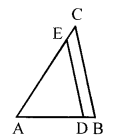Question 4.
Find the distance of origin from the point P(3, -2). (NTSE2012)

Question 5.
Find the 9th term from the end (towards the first term) of the AP. 5,9,13,……, 185

Question 6.
Find the discriminant ofthe quadratic equation 3$\sqrt { 3 }$x2 +10x + $\sqrt { 3 }$ = 0

Section-B

Question 7.
Show that 5- $\sqrt { 3 }$ is not irrational.

Question 8.
Cards marked with numbers 13,14,15,…….., 60 are placed in a box and mixed thoroughly. One card is
drawn at random from the box. Find the probability that the number on the drawn card is
(i) divisible by 5
(ii) a number which is a perfect square

Question 9.
If p, q, r are in A.P. then find the value of p3 +r3 – 8q3.

Question 10.
For all real values of c, the pair of equations
x – 2y = 8
5x- 10y = c
have a unique solution. Justify whether it is true or false.

Question 11.
If the coordinates of points A and B are (-2, -2) and (2, -4) respectively, find the coordinates of P such that AP= [latex s=2]\frac { 3 }{ 7 } [/latex]AB, where Plies on the line segment AB.

Question 12.
A piggy bank contains hundred 50p coins, fifty ₹ 1 coins, twenty ₹ 2 coins and ten ₹ 5 coins. If it is equally likely that one of the coins will fall out when the bank is turned upside down, what is the probability that the coin (i) will be a 50 p coin? (ii) will not be a ₹ 5 coin?

Section-C

Question 13.
Show that any positive odd integer is of the form 6q – 1 or 6q + 5, where q is some integer.

Question 14.
What must be subtracted from x3 – 6x2 – 15x + 80, so that the result is exactly divisible by x2 + x— 12.

Question 15.
If a cos θ + b sinθ = m and a sin θ – b cosθ = n, prove that a2 + b2 = m2 + n2
OR
If x = r sin A cos C, y = r sin A sin C, z = r cos A, Prove that : r2 = x2+ y2+ z2

Question 16.
Calculate the area of the shaded portion.
The quadrants shown in the figure are each of radius 7 cm. [Take π = [latex s=2]\frac { 22 }{ 7 } [/latex]]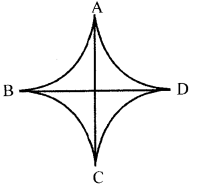Question 17.
If mean of the following frequency distribution is 7.5, then find the value of p.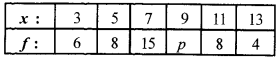Question 18.
An iron pillar has some parts in the form of a right circular cylinder and remaining in the form of a right circular cone. The radius of the base of each cone and cylinder is 8 cm. The cylindrical part is 240 cm high and the conical part is 36 cm high. Find the weight of the Pillar if one cubic cm. of iron weight 7.8 gms.
OR
A cylinder whose height is two-third of its diameter has the same volume as a sphere of radius 4 cm. Calculate the radius of the base of the cylinder.

Question 19.
The numerator of a fraction is 4 less than the denominator. If the numerator is decreased by 2 and the denominator is increased by 1, then the denominator is eight times the numerator. The fraction is [latex s=2]\frac { a }{ b } [/latex] . Find [latex s=2]\frac { a+b }{ 2 } [/latex]

Question 20.
If the diagonal BD of a quadrilateral ABCD bisects both ∠B and ∠D then show that [latex s=2]\frac { AB }{ BC } [/latex] = [latex s=2]\frac { AD }{ CD } [/latex]
OR
In an equilateral triangle ABC, AD is drawn perpendicular to BC. Prove that 4 AD2 = 3BD2

Question 21.
Find the values of K, so that the area of the triangle with vertices (1, -1), (-4,2k) and (-k, -5) is 24 sq. units.
OR
If the point P (x, y) is equidistant from the points A (a + b, b – a) and B (a – b, a + b). Prove that bx = ay.

Question 22.
If from an external point P of a circle with centre 0, two tangents PQ and PR are drawn such that ∠QPR =120°, prove that 2PQ = PO.

Section-D

Question 23.
The ratio of the sums of the first m and first n terms of an A.P. is m2: n2. Show that the ratio of its mth and nth terms is (2m -1): (2n – 1).

Question 24.
If the areas of two similar triangles are equal, prove that they are congruent.
OR
If the corresponding sides of two triangles are proportional (i.e., in the same ratio), then prove that their corresponding angles are equal and hence the two triangles are similar.

Question 25.
At a point, A, 20 metres above the level of water in a lake, the angle of elevation of a cloud is 30°. The angle of depression of the reflection of the cloud in the lake, at A is 60°. Find the distance of the cloud from A.
OR
A bird is sitting on the top of an 80 m high tree. From a point on the ground, the angle of elevation of the bird is 45°. The bird flies away horizontally in such a way that it remained at a constant height from the ground. After 2 seconds, the angle of elevation of the bird from the same point is 30°. Find the speed of flying of the bird.
(Take $\sqrt { 3 }$ =1.732)

Question 26.
A semicircular sheet of metal of diameter 28 cm is bent into an open conical cup. Find the depth and capacity of the cup.

Question 27.
If θ be an acute angle such that tan2 θ = 1 – a2, then find the value of {sec θ + tan3 θ cosec θ}2

Question 28.
Solve the quadratic equation (x2 – 5x)2 – 7(x2 – 5x) + 6 = 0.

Question 29.
Construct a triangle with sides 5 cm, 5.5 cm and 6.5 cm. Now construct another triangle, whose sides are [latex s=2]\frac { 3 }{ 5 } [/latex] times the corresponding sides of the given triangle.

Question 30.
The following table gives the ages in years of militants operating in a certain area of a country: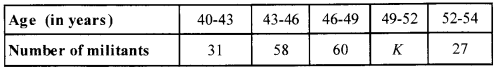If the mean of the above distribution is 47.2, find how many militants in the age groups 49-52 are active in the area?
OR
One hundred students took an examination. Compute values ofx and y from the following data if mean is 42.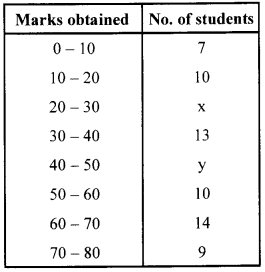Solution 1.
3 × 5 ×7+7 = 7 (3 × 5 + 1) = 7 (16), which has more than two factors.
∴ It is a composite number. (1)

Solution 2.
sinA= [latex s=2]\frac { 1 }{ \sqrt { 2 } } [/latex] ⇒ A=45°

and tan B = 1 ⇒ B = 45° (1/2)
∴ sin (A + B) = sin (45° + 45°) ⇒ sin 90° = 1 Hence Proved. (1/2)

Solution 3.
In ∆ABC, we have DE || BC
∴ [latex s=2]\frac { AD }{ DB } [/latex] = [latex s=2]\frac { AE }{ EC } [/latex] By Thale’s Theorem] (1/2)

⇒ [latex s=2]\frac { x }{ x-2 } [/latex] = [latex s=2]\frac { x+2 }{ x-1 } [/latex]
⇒x(x-1) = (x-2)(x +2) ⇒ x2 – x = x2 – 4 ⇒x = 4 (1/2)

Solution 4.
Distance = $\sqrt { { (0-3) }^{ 2 }+{ (0+2) }^{ 2 } } =\sqrt { 9+4 } =\sqrt { 13 }$ (1)

Solution 5.
Common difference of the AP = d = 9 – 5 = 4
Last term of the AP = l= 185 (1/2)
Since the nth term from the end of an AP is given by l – (n – 1 )d.
So, the 9th term from the end is = 185 – (9 – 1)4 = 185 – 32= 153 (1/2)

Solution 6.
D = b2 – 4ac = (10)2 – 4(3$\sqrt { 3 }$ )($\sqrt { 3 }$) = 100 – 36 = 64 (1)

(Section-B)

Solution 7.
If 5 – $\sqrt { 3 }$ is not irrational.
Let 5 – $\sqrt { 3 }$ = [latex s=2]\frac { p }{ q } [/latex] [p and q are integers, q ≠ 0] (1/2)
⇒[latex s=2]\frac { 5q-p }{ q } [/latex] = $\sqrt { 3 }$ ⇒ [latex s=2]\frac { 5q-p }{ 3 } [/latex] is rational number  (1)
So, $\sqrt { 3 }$ should be rational.
But $\sqrt { 3 }$ is irrational, it cannot be equal to rational number, Hence 5 -$\sqrt { 3 }$ is irrational. (1/2)

Solution 8.
Total no. of cards = 60-12 = 48
Numbers are 13,14,15,16,……….,60.
(i) Numbers divisible by 5 are 15, 20,25, 30, 35, 40, 45, 50, 55, 60. (1/2)
∴ Favourable outcomes = 10
∴ P(no. is divisible by 5) = [latex s=2]\frac { 10 }{ 48 } [/latex] = [latex s=2]\frac { 5 }{ 24 } [/latex] (1/2)
(ii) Perfect square numbers are 16,25,36,49 (1/2)
∴ Ptperfect square) = [latex s=2]\frac { 4 }{ 48 } [/latex] = [latex s=2]\frac { 1 }{ 12 } [/latex] (1/2)

Solution 9.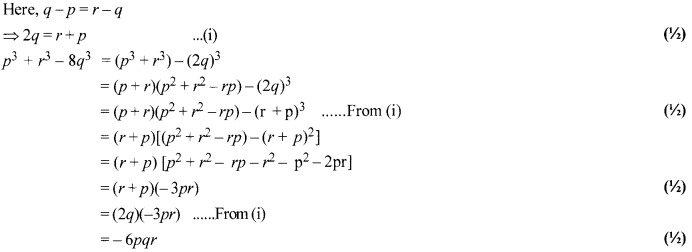Solution 10.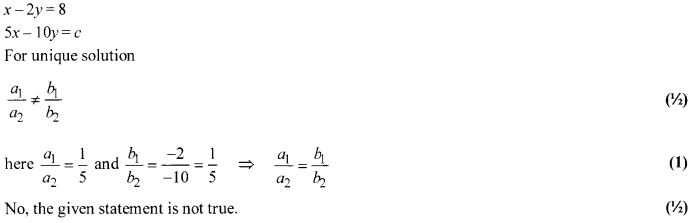Solution 11.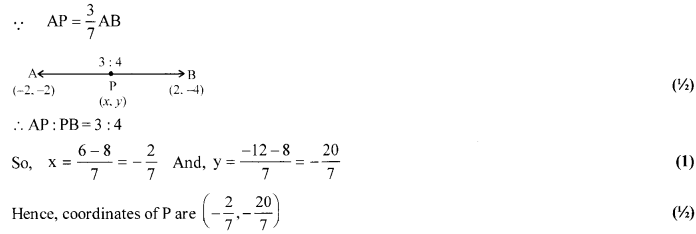Solution 12.
Number of 50 p coins = 100
Number of ₹ 1 coins = 50
Number of ₹ 2 coins = 20
Number of ₹ 5 coins = 10
Total number of coins = 180 (1/2)
Then (i) P (50 p coin) = [latex s=2]\frac { 100 }{ 180 } [/latex] = [latex s=2]\frac { 5 }{ 9 } [/latex]
(ii) P (not a ₹ 5 coin) = 1 – P (a ₹ 5 coin) (1/2)
= 1- [latex s=2]\frac { 10 }{ 180 } [/latex] = 1- [latex s=2]\frac { 1 }{ 18 } [/latex] = [latex s=2]\frac { 17 }{ 18 } [/latex] (1)

(Section-C)

Solution 13.
Let a be any positive integer and b = 6
Then by Euclid’s algorithm, a = bq + r, o < r < b (1/2)
Wehavea = 6q+r   …(1)
Since 0 ≤ r < 6, So, r = 0,1,2,3,4,5
∴ From(1), forr = 0, a = 6q
forr = 1, a = 6q + 1
forr = 2, a = 6q + 2
forr = 3, a = 6q + 3
forr = 4, a = 6q + 4
forr = 5, a = 6q + 5   (1/2)
Since 6q is divisible by 2,
∴ 6q is even. (1)
6q + 1 is not divisible by 2. So, 6q + 1 l is odd.
6q + 2 is divisible by 2
∴ 6q + 2 is even. (1)
6q + 3 is not divisible by 2. So, 6q + 3 is odd.
6q + 4 is divisible by 2.
∴ 6q + 4 is even.
6q + 5 is not divisible by 2. So, 6q + 5 is odd. (1/2)
So, we see that 6q, 6q + 2, 6q + 4 are even. Since the number which are not divisible by 2 are odd integer.
∴ 6q +1, 6q + 3, 6q + 5 are odd integer. Hence any positive odd integer is ofthe form 6q + 1, or 6q + 3, or 6q + 5, where q is some integer. (1/2)

Solution 14.
Let (ax + b) be subtracted from given polynomial p(x), so that it is exactly divisible by x2 + x – 12 Then, .
q (x) = x3-6x2 – 15x + 80 – (ax + b)
= x3 -6x2 – (15 + a)x + (80 -b) (1/2)
∵ Dividend = Divisor × quotient + remainder (A)
But remainder will be zero.
∴ Dividend = Divisor x quotient
q (x) = (x2 + x – 12) x quotient
∴q(x) = x3-6x2-(15+a)x + (80 – b) (1/2)
Also, x(x2 +x- 12)-7(x2 +x- 12)
= x3 + x2 – 7x2 – 12x – 7x + 84
= x3 – 6x2 – 19x + 84 (1)
Hence, x3 – 6x2 – 19x + 84 = x3 – 6x2 – (15 + a)x + (80 – b)
– 15 – a = – 19 ⇒ a = + 4 and 80 – b = 84 ⇒ b = – 4 (1)
Hence, ifinp (x) we subtracted 4x – 4 = (ax + b), then it is exactly divisible by x2 + x – 12

Solution 15.
m = a cos θ + b sin θ
(m)2= (a cos θ + b sin θ)2
m2 = a2 cos2 θ + bs2 sin2θ + 2ab cos θ sin θ …(i) (1)
also, n = a sin θ – b cos θ ;
(n)2 = (a sin θ – b cos θ)2
n2 = a2sin2 θ + b2 cos2 θ – 2ab cos θ sin θ …(ii) (1)
On adding equation (i) and (ii), we get
m2 + n2 = a2 cos2 θ + b2 sin2 θ + a2 sin2 θ + b2 cos2 θ
2(cos2 θ + sin2 θ) + b2(sin2θ + cos2 θ) (1)
m2 + n2 = a2 + b2
Hence proved.
OR
x = r sin A cos C, y = r sin A sin C and z = r cos A
x2+ y2+ z2 = r2sin2A cos2C + =(r2 sin2A ) (cos2C + sin2C) +r2 cos2A  (1)
= r2 sin2 A(1) + r2 cos2 A  (1/2)
= r2(sin2 A + cos2 A) =r2 Hence Proved. (1/2)

Solution 16.
Draw square PQRS as shown in the figure given below.
Here PQ= QR = PS= RS = 14 cm (1)
Area of the shaded portion = Area of the square PQRS – Area of four equal quadrants (1)
= 14 × 14 – 4 × [latex s=2]\frac { 1 }{ 4 } [/latex]π × (7)2 =196 – [latex s=2]\frac { 22 }{ 7 } [/latex] 7 ×7
= 196-154 =42 cm2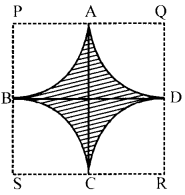Solution 17.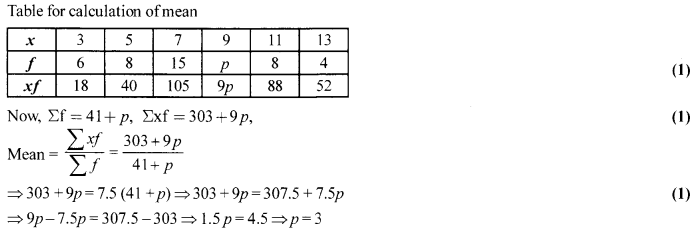Solution 18.
V of cylindrical part = π(8)2 (240) = 64π × 240 (1/2)
V of conical part = [latex s=2]\frac { 1 }{ 3 } [/latex]π × 64 × 36 =64π(12) (1/2)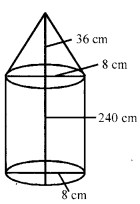Total volume of iron = 64π(240 + 12) = 64×[latex s=2]\frac { 22 }{ 7 } [/latex] ×252 = 64 × 22× 36 cm3 (1)
∴ Total weight ofpillar = (64) (22) (36) × 7.8 = 395366.4 gms = 395.3664 kg (1)
OR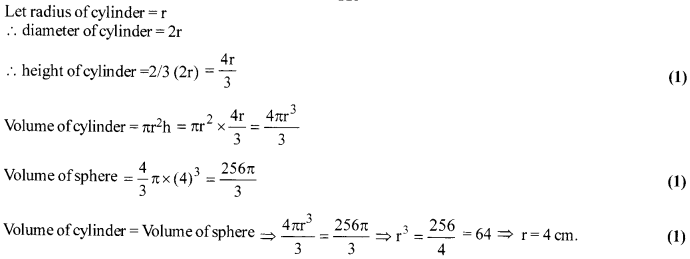Solution 19.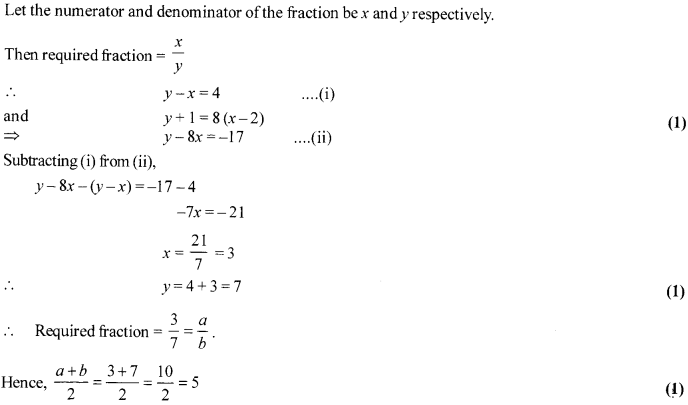Solution 20.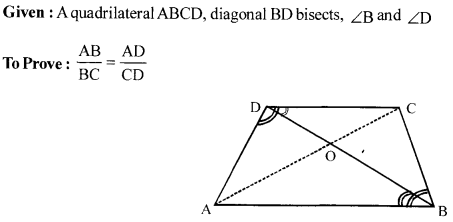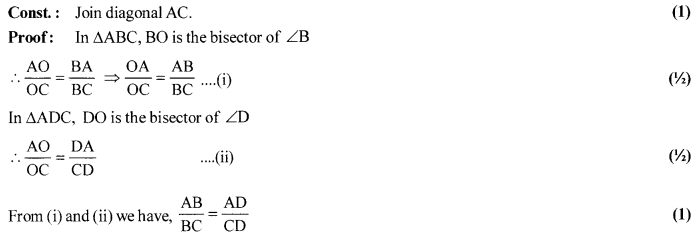OR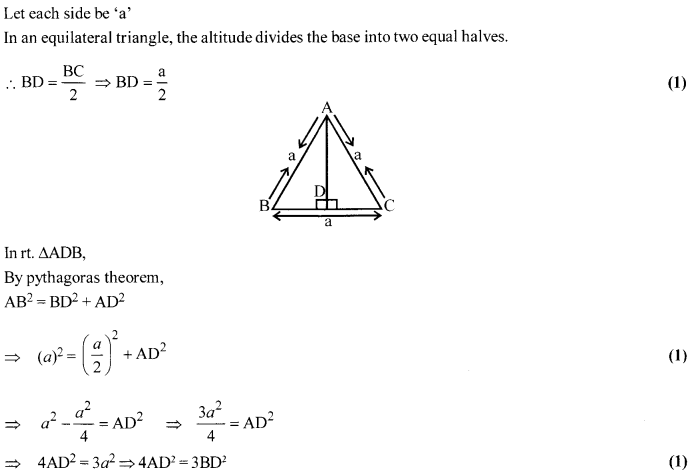Solution 21.
∵ Area of the triangle = 24 sq. units (1)
∴[latex s=2]\frac { 1 }{ 2 } [/latex][1(2k + 5)-4(-5 + 1)-k(-1-2k)] = 24
So, 2k2 + 3k- 27 = 0 ⇒ (2k + 9) (k – 3) = 0
After solving we get k = 3, -[latex s=2]\frac { 9 }{ 2 } [/latex] (1)
OR
The point P (x, y) is equidistant form the points A (a + b, b – a) and B (a – b, a + b)
∴ PA= PB
⇒ $\sqrt { { (a+b-x) }^{ 2 }+{ (b-a-y) }^{ 2 } } =\sqrt { { (a-b-x) }^{ 2 }+{ (a+b-y) }^{ 2 } }$ (1/2)
⇒ (a + b – x)2 + (b – a – y)2 = (a – b – x)2 + (a + b – y)2 (1/2)
⇒ (a + b – x)2 – (a – b – x)2 = (a + b – y)2 – (b – a – y)2 (1/2)
⇒ (a + b-x + a-b-x) (a + b-x-a + b + x) = (a + b-y + b-a-y) (a + b-y-b+ a + y) (1/2)
⇒ (2a-2x)(2b) = (2b-2y)(2a)
⇒ (a – x) b = (b – y) a
⇒ ab – bx = ab – ay ⇒ bx = ay (1)

Solution 22.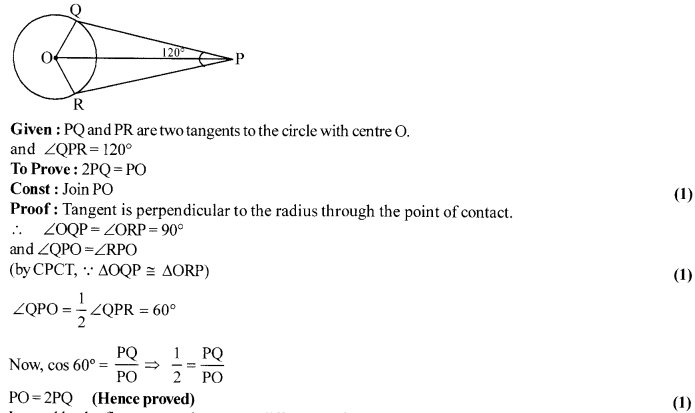Section-D

Solution 23.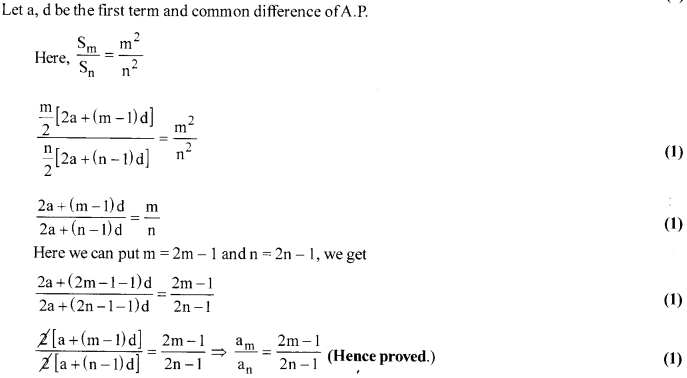Solution 24.
Let ∆ABC ~ ∆PQR and.
area (∆ABC) = area (∆PQR) (Given) (1/2)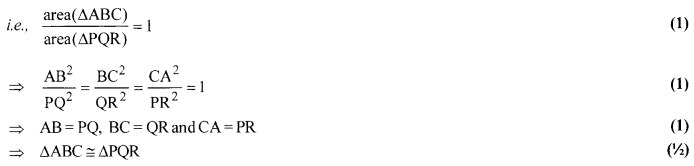OR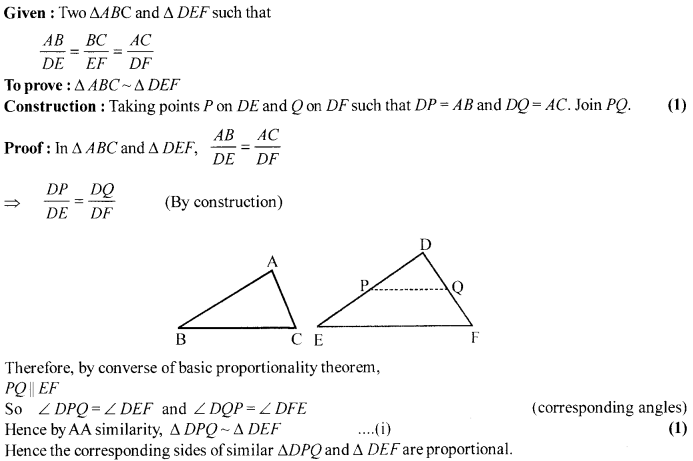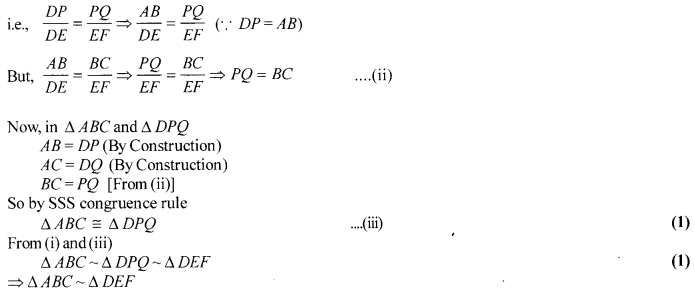Solution 25.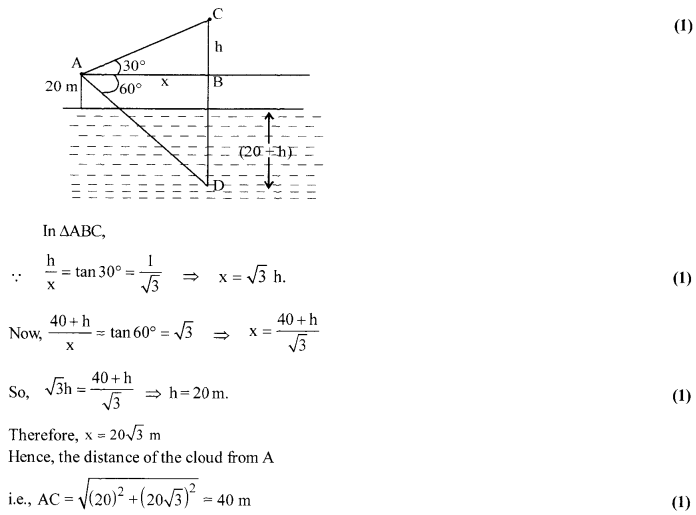OR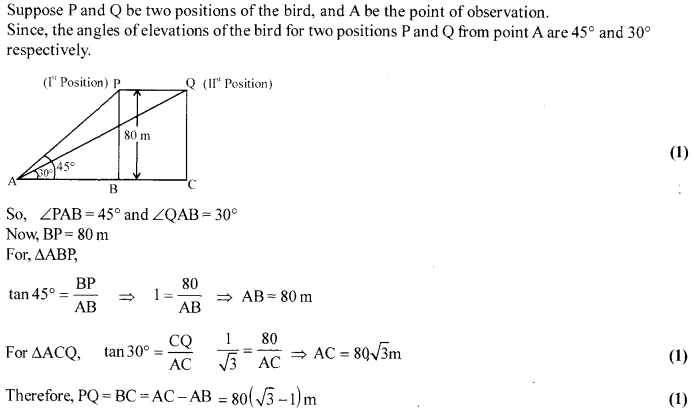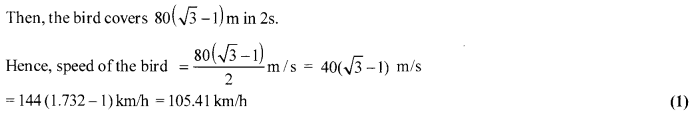Solution 26.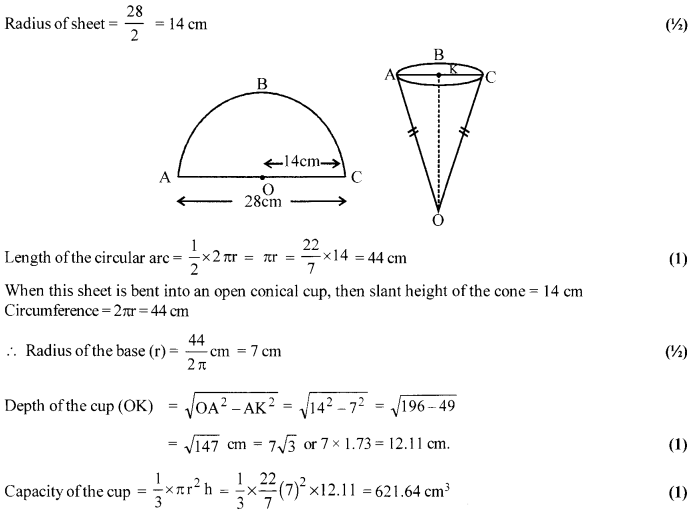Solution 27.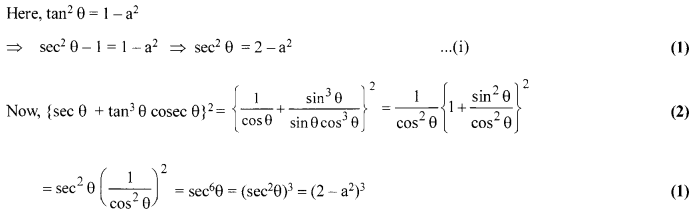Solution 28.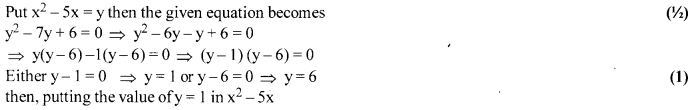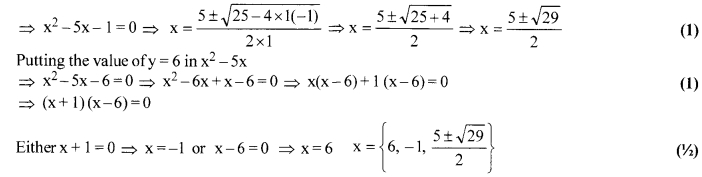Solution 29.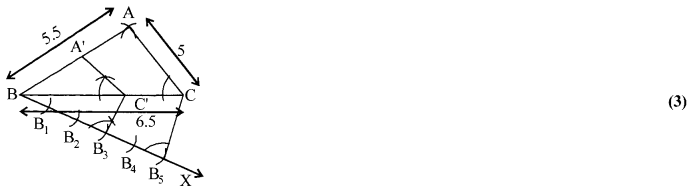Steps of construction
1. Draw a line segment BC = 6.5 cm.
2. Take B as a centre and mark an arc of length 5.5 cm above BC.
3. Similarly, take C as a centre and cut an arc above BC of length 5 cm which cuts the previous arc at point A.
4. Join AB and AC. The required triangle is formed i.e., ∆ABC
5. Draw a ray BX below BC.
6. Mark equal arcs B1, B2, B3, B4 and B5 on BX such that BB1 = B1B2 = B2B3 = B3B4 = B4B5.
7. Join B5C and draw a line || to B5C from point B3 which cuts BC at C’.
8. Drawaline||toACfromC’whichcutsAB at A’. (1)
9. Now, ∆A’BC’ is the required triangle.

Solution 30.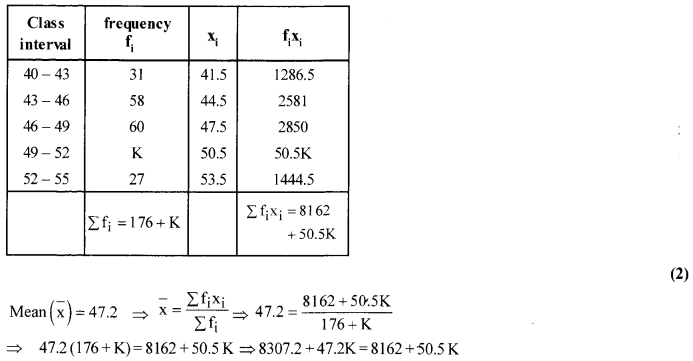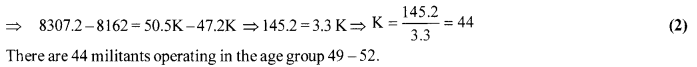OR